•
百度
•谷歌
•
360搜索
•
搜狗搜索
•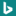必应
•
中国搜索
•Wikipedia
•搜微信
•
搜知乎
•
找快递
•
视频
•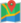地图
•高德地图
•百度地图
•
谷歌地图
•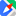腾讯地图
•搜狗地图
•必应地图
•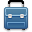旅游
•
蚂蜂窝
•
穷游
•猫途鹰
•
缤客
•
乐途
•爱彼迎
•途牛
•
有道词典
•
找歌谱
•
Visual Hunt
•
音乐搜索解析
•小程序
•
世图
•图标
•GIF动图
•搜狗表情
•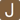电子书
•Wolfram Alpha
•
小白盘
•
动漫资源
•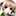动漫 BT
•
ACG搜
•百度识图
•
特殊符号识别
•求字体
•Visual Hunt
•人物关系
•
姓氏家谱
•汉典
•
象形文字
•
国学
•古文献
•MBA智库
•
字幕
•
哔哩哔哩
•比一比价
•
商品历史价格
•数码电器比价
•
美食美味
•狗狗识别器
•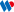专利检索
•
易车
•
求解答
•
工商信息
•
海关
•
声音
•
国家标准
•
开源代码
•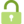开源文献
•海关
•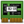电子元件数据
• 搜索引擎（Search Engine）是指根据一定的策略、运用特定的计算机程序从互联网上搜集信息，在对信息进行组织和处理后，为用户提供检索服务，将用户检索相关的信息展示给用户的系统。搜索引擎包括全文索引、目录索引、元搜索引擎、垂直搜索引擎、集合式搜索引擎、门户搜索引擎与免费链接列表等。